## What will you build

You will overide some of the methods that are implements in every Object in Java

## What You'll Learn

• Why overriding a method
• How to override a method

## What will you need

• Atom
• Java JDK installed
• Gitbash or any type of terminal

Overriding in Java is the action to redefine a method that have already been defined in a super class. It is allowing to modify the behaviour of an object.

Really important: The overriding method has the same name, number and type of parameters and return type as the method that it overrides.

In java, every classe is an Object, and they all inherit from Object in Java.

By consequence, all objects inherit from some basic methods:

• clone()
• equals()
• finalize()
• getClass()
• hashCode()
• notify()
• notifyAll()
• toString()
• wait()
• wait(long timeout)
• wait(long timeout, int nanos)

All objects can invoke these methods. However, you cannot invoke these methods in primitive.

We will see inheritance in depth in other codelabs.

In this codelabs, we will see only the method equals, hashcode and toString. When you override these methods, it is important to follow the convention. If you are not following the convention, you should indicate it in your documentation, as if not, the developper using your class will have some expectation that are not fulfil.

To see more about these methods and see the convention

The toString method returns a String representation of the object. Lets try it on the Tea Class defined in previous codelabs. I simplify it for the purpose of the demonstration.

``````public class Tea {

private String typeTea;
private double price;

public Tea(String typeTea, double price){
this.typeTea = typeTea;
this.price=price;
}

public double getPrice(){
return price;
}

public String getTypeTea(){
return typeTea;
}
}```

The class to test the Tea class

```java
public class TestToString {
public static void main (String[] args) {
Tea tea1 = new Tea("English Breakfast",10.0);
System.out.println(tea1.toString());
}
}
``````

Running this code you should obtain this:It is not what we would like, this displays a string consisting of the name of the class (Tea) followed with the ‘@', and the unsigned hexadecimal representation of the hash code of the object.

What we want is to return a simple "textual representation" of this object, and that is readable for a person. To be able to do that, we will override the method to String.

To override a method, we will implement this method in the class Tea.

``````    @Override
public String toString () {
return "Tea "+getTypeTea()+ " price "+getPrice();
}
``````

You have some simple rules to follow for this method, it needs to return a String, and need to be called toString, as we override the method toString(). The rest of the method is up to you, but should be representing the information inside your class.

The annotation "@Override" is not mandatory but indicated to the compiler that you are overriding the method. If the method that you are overriding doesn't exist in the super class of your class, it will raise an error at compile time.

The result of adding this method in the class Tea: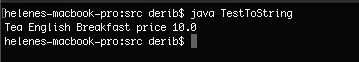As we saw in the codelabs on equality, to test the equality between to object, we need to use the method equals(). This include String, you should never use the "==" to test the equality of the value of the String.

If we don't override the method equals on a class, and we want to test it the content of a class is the same, it will not work.

``````public class TestToString {
public static void main (String[] args) {
Tea tea1 = new Tea("English Breakfast",10.0);
System.out.println(tea1.toString());
Tea tea2 = new Tea("English Breakfast",10.0);
System.out.println(tea2.toString());
if(tea1.equals(tea2)){
System.out.println("This is the same Tea");
}else{
System.out.println("This is not the same Tea");
}
}
}
``````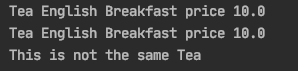As you can observe, the result is that my two tea that have the same values for the name and the price are not the same. In term of instance of object, this is true, it is not the same instance, I did created two instances of the object Tea. However, if I think as a person, it should be the same, and the developper using my class will certainly think that if two Teas have the same values, they should be the same.

Overriding the method equals will allows this.

To override the method equals, you should follow the convention given by Java, and follow a certain logic. You can find it here more explanation.

The equals method implements an equivalence relation on non-null object references:

• It is reflexive: for any non-null reference value x, x.equals(x) should return true.
• It is symmetric: for any non-null reference values x and y, x.equals(y) should return true if and only if y.equals(x) returns true.
• It is transitive: for any non-null reference values x, y, and z, if x.equals(y) returns true and y.equals(z) returns true, - then x.equals(z) should return true.
• It is consistent: for any non-null reference values x and y, multiple invocations of x.equals(y) consistently return true or consistently return false, provided no information used in equals comparisons on the objects is modified.
• For any non-null reference value x, x.equals(null) should return false.

When you override the method, you should follow these logical rules.

``````    @Override
public boolean equals (Object o) {
if (this == o) return true;
if (!(o instanceof Tea)) return false;

Tea tea = (Tea) o;
if (Double.compare(tea.getPrice(), getPrice()) != 0) return false;
return getTypeTea().equals(tea.getTypeTea());
}
``````

If this method is correctly override in the class Tea, now I am running the same code again

``````public class TestToString {
public static void main (String[] args) {
Tea tea1 = new Tea("English Breakfast",10.0);
System.out.println(tea1.toString());
Tea tea2 = new Tea("English Breakfast",10.0);
System.out.println(tea2.toString());
if(tea1.equals(tea2)){
System.out.println("This is the same Tea");
}else{
System.out.println("This is not the same Tea");
}
}
}
``````

And this time it is working as expected: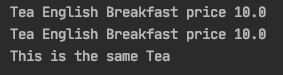When you override the method equals, it is normally necessery to override the method hashCode(). The contract states that when an object is equal, the hashcode should be equal.

If you don't override it this could lead to some unexpected result.

Let's try it, for the moment the hashCode is not override.

If I implement a Set of Tea. I want to have only one instance of Tea that is kept in the Set.

The class Tea

``````public class Tea{

private String typeTea;
private double price;

public Tea(String typeTea, double price){
this.typeTea = typeTea;
this.price=price;
}

public void setPrice(double price){
this.price = price;
}

public double getPrice(){
return price;
}

public String getTypeTea(){
return typeTea;
}

@Override
public String toString () {
return "Tea "+getTypeTea()+ " price "+getPrice();
}

@Override
public boolean equals (Object o) {
if (this == o) return true;
if (!(o instanceof Tea)) return false;

Tea tea = (Tea) o;
//if (((Double)getPrice()).compareTo(getPrice())!= 0) return false;;
if (Double.compare(tea.getPrice(), getPrice()) != 0) return false;
return getTypeTea().equals(tea.getTypeTea());
}
}
``````

As usual, I am using another class to test my class Tea.

``````import java.util.HashSet;
import java.util.Set;

public class TestToString {
public static void main (String[] args) throws CloneNotSupportedException {
Tea tea1 = new Tea("English Breakfast",10.0);
Tea tea2 = new Tea("English breakfast",10.0);
Set<Tea> teas = new HashSet<>();
for(Tea t: teas){
System.out.println(t.toString());
}
}
}
``````

The result of this is: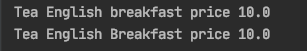As you can observe, we have two instances of the class Tea. Logically, we would like to have only one instance of this tea, as the value are the same.

To be able to do that, we need to override the hashCode method, as the class HashSet compare the HashCode of the instance to determine if this is the same object. This is one possibility to override the hashCode method. The better the hashing alorithm is, the better the performance of the hash table will be.

`````` @Override
public int hashCode() {
int hash = 17;
hash = (int) (31* hash + price);
hash = 31 * hash + (typeTea == null ?0:typeTea.hashCode());
return hash;
}
``````

The result of this is now correct: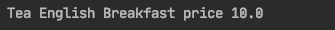In addition, one of the reason to always override hashCode method, is that it is expected by any developper of Java.

See documentation there

• How to override a method
• Why to override a method
• Why to override HashCode when you have override equals.

You can find the code for this example in https://git.cardiff.ac.uk/ASE_GROUP_2020/code_for_codelabs.git